One-proportion z test in R

For quite a while, I’ve been confused by the behavior of the prop.test function in R: 1) the p-values always seem to be slightly off, and 2) the confidence intervals never seem to be symmetric around my estimate of the population proportion. Today, I decided I was going to figure out what was up… and whether I should trust my own analytical calculations for this test or what I get in R. I started by checking R Bloggers to see if anyone had explored this problem, but I only found one post about the two proportion z-test, and found none focusing on the one proportion z-test.

Here’s the essence of the test: you observe x subjects out of a sample size of n observations. Perhaps you just want to create a confidence interval to get a sense of where the true population proportion lies. Or maybe, you’d like to test the null hypothesis that the true population proportion is some value p0, against the alternative that it is really greater than, less than, or not equal to that value.

To do this, you can use the normal approximation to the binomial distribution to calculate a test statistic z. The estimated population proportion, p-hat, is just x/n. You have to find the standard error (SE) of your estimate as well to get the value of z: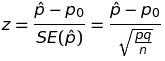The confidence interval is constructed from the estimated population proportion, and the margin of error, which is determined by the standard error of the estimate and a scaling factor (z*) that establishes the width of the confidence interval: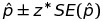Here’s an example. Let’s say I know that nationally, 90% of home buyers would select a home with energy efficient features, even paying a premium of 2-3%, to reduce their utility costs long term. As the hypothetical president of an engineering firm that installs these energy efficient features, I want to know if less than 90% of buyers in my local area feel this way (because if so, I might want to launch an education campaign to help my business). I randomly sample 360 potential buyers and find that 312 of them would indeed select a home with energy efficient features.

I want to test my sample against the null hypothesis that the true population proportion is 0.90, with the alternative that the proportion is less than 0.90. I use a estimated population proportion (p-hat) of 312/360=0.867, and using the equations above, find that my test statistic z turns out to be -2.108, with a corresponding p-value of 0.0175. I reject the null hypothesis that the true population proportion is 0.90 in favor of the alternative, and start making plans to launch my education program.

(I don’t get the same values when I use prop.test. But later in this post, I’ll show you why I shouldn’t have expected that in the first place!!)

I wrote an R function to automate my z test (and also, to double check my calculations):

z.test <- function(x,n,p=NULL,conf.level=0.95,alternative="less") {
ts.z <- NULL
cint <- NULL
p.val <- NULL
phat <- x/n
qhat <- 1 - phat
# If you have p0 from the population or H0, use it.
# Otherwise, use phat and qhat to find SE.phat:
if(length(p) > 0) {
q <- 1-p
SE.phat <- sqrt((p*q)/n)
ts.z <- (phat - p)/SE.phat
p.val <- pnorm(ts.z)
if(alternative=="two.sided") {
p.val <- p.val * 2
}
if(alternative=="greater") {
p.val <- 1 - p.val
}
} else {
# If all you have is your sample, use phat to find
# SE.phat, and don't run the hypothesis test:
SE.phat <- sqrt((phat*qhat)/n)
}
cint <- phat + c(
-1*((qnorm(((1 - conf.level)/2) + conf.level))*SE.phat),
((qnorm(((1 - conf.level)/2) + conf.level))*SE.phat) )
return(list(estimate=phat,ts.z=ts.z,p.val=p.val,cint=cint))
}

When I run it on my example above, I get what I expect:

> z.test(312,360,p=0.9)
\$estimate
 0.867

\$ts.z
 -2.11

\$p.val
 0.0175

\$cint
 0.836 0.898

But when I run prop.test, this isn’t what I see at all!

> prop.test(312,360,p=0.9)

1-sample proportions test with continuity correction

data: 312 out of 360, null probability 0.9
X-squared = 4.08, df = 1, p-value = 0.04335
alternative hypothesis: true p is not equal to 0.9
95 percent confidence interval:
0.826 0.899
sample estimates:
p
0.867

Why are there differences? Here’s what I found out:

• The prop.test function doesn’t even do a z test. It does a Chi-square test, based on there being one categorical variable with two states (success and failure)! Which I should have noticed immediately from the line that starts with “X-squared” 🙂
• The Yates continuity correction, which adjusts for the differences that come from using a normal approximation to the binomial distribution, is also automatically applied. This removes 0.5/n from the lower limit of the confidence interval, and adds 0.5/n to the upper limit.
• Most notably, I was really disturbed to see that the confidence interval given by prop.test wasn’t even centered around the sample estimate, p-hat. Oh no! But again, this is no concern, since prop.test uses the Wilson score interval to construct the confidence interval. This results in an asymmetric, but presumably more accurate (with respect to the real population) confidence interval.

Moral of the story? There are many ways to test an observed proportion against a standard, target, or recommended value. If you’re doing research, and especially if you’re using R, be sure to know what’s going on under the hood before you report your results.

(Other moral? Try binom.test.)

A Linear Congruential Generator (LCG) in R

In my simulation classes, we talk about how to generate random numbers. One of the techniques we talk about is the Linear Congruential Generator (LCG). Starting with a seed, the LCG produces the first number in the sequence, and then uses that value to generate the second one. The second value is used to generate the third, the third to generate the fourth, and so on. The equation looks like this: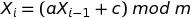where a is a multiplier, c is a shift, and m is a modulus. (Modulus just means “find me the remainder when you divide the stuff to the left of the mod operator by the stuff to the right”. Fortunately there is an easy way to do this in R: a mod b is expressed as a %% b.) When you use the LCG to generate a stream of random numbers, they will always be between 0 and (m-1), and the sequence will repeat every m occurrences. When you take the sequence of values x and divide it by the mod m, you get uniformly distributed random numbers between 0 and 1 (but never quite hitting 1). The LCG is a pseudorandom number generator because after a while, the sequence in the stream of numbers will begin to repeat.

I wrote an exam last week with some LCG computations on it, but then I got lazy and didn’t want to do the manual calculations… so I wrote a function in R. Here it is. It returns two different vectors: the collection of numbers between 0 and (m – 1) that the LCG produces, and the collection of uniformly distributed random numbers between 0 and 1 that we get by dividing the first collection by m:

lcg <- function(a,c,m,run.length,seed) {
x <- rep(0,run.length)
x <- seed
for (i in 1:(run.length-1)) {
x[i+1] <- (a * x[i] + c) %% m
}
U <- x/m # scale all of the x's to
# produce uniformly distributed
# random numbers between [0,1)
return(list(x=x,U=U))
}

So for example, if your LCG looks like this: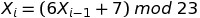Then, after loading in the function above, you could choose a seed (say, 5) and generate a stream of 20 random numbers:

> z <- lcg(6,7,23,20,5)
> z
\$x
 5 14 22 1 13 16 11 4 8 9 15 5 14 22 1 13 16 11
 4 8

\$U
 0.21739130 0.60869565 0.95652174 0.04347826 0.56521739
 0.69565217 0.47826087 0.17391304 0.34782609 0.39130435
 0.65217391 0.21739130 0.60869565 0.95652174 0.04347826
 0.56521739 0.69565217 0.47826087 0.17391304 0.34782609

The values contained within z\$x are the numbers that the LCG produces, and the values contained within z\$U are the values of z\$x scaled to fall between 0 and 1. Both z\$x and z\$U are uniformly distributed, although the pattern will become more apparent as you generate more and more random numbers in your stream. Here’s what they look like with 10000 numbers in the stream:

> z <- lcg(6,7,23,10000,5)
> par(mfrow=c(1,2))
> hist(z\$x,col="red")
> hist(z\$U,col="purple")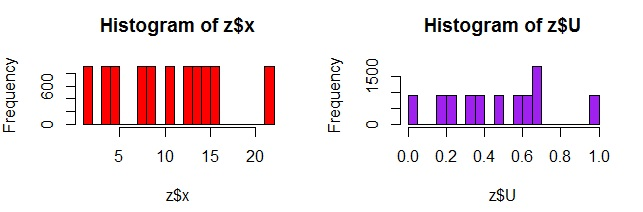But how good is your random number generator? First, you know you want a large (and preferably odd valued mod). That way, the numbers will not repeat so quickly. But there are also considerations dealing with what multiplier and shift you should use. I like checking out the quality of my random number generator by plotting how they move in sequence around a two dimensional grid: the better the random number generator, the more filling of the space you will see.

You can play around with these plots to find a “good” random number generator:

> z1 <- lcg(6,7,23,2000,5) # "Bad" LCG
> z2 <- lcg(1093,18257,86436,2000,12) # "Good" LCG
> par(mfrow=c(1,2))
> plot( z1\$U[1:(length(z1\$U)-1)], z1\$U[2:length(z1\$U)] , main="Bad")
> plot( z2\$U[1:(length(z2\$U)-1)], z2\$U[2:length(z2\$U)] , main="Good")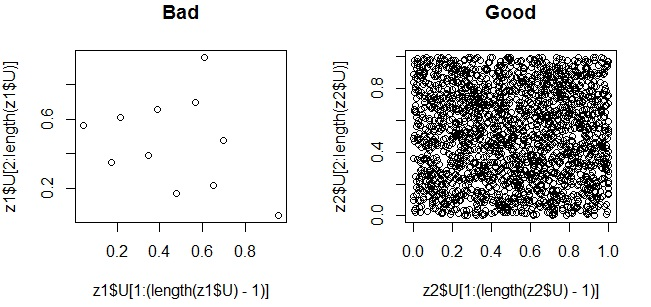Although there are many heuristics you can use to avoid creating a “bad” random number generator, it’s really difficult to design a “good” one. People make careers out of this. I don’t claim to be an expert, I just know how to test to see if a random number generator is suitable for my simulations. (I also really like the handout at http://www.sci.csueastbay.edu/~btrumbo/Stat3401/Hand3401/CongGenIntroB.pdf that explains this graphing approach more, and also talks about how you have to look at patterns in three dimensions as well. When I was searching around for a “good LCG that would give me a nice graph” I found this handout.)

Typing x-bar, y-bar, p-hat, q-hat, and all that! In Microsoft Word (& Excel)

I use Microsoft Word to prepare documents. I do not like Microsoft Equation Editor. And I have to type equations and expressions not often (like every sentence or every other sentence), but definitely regularly. This has led me to apply what I like to call “agile shortcuts” — basically, I’ll write down the equation in my own handwriting, take a picture of it, and then use a paint program to crop and clean up my equation before inserting it into my document. This works nicely, and even though some people might think it’s a kludge, I kind of like the ability to retain the personality of my own handwriting in my technical documents.

However, I don’t want to be embedding images if all I have to do is make reference to a variable within a paragraph of text… and I’ve never had a good solution. UNTIL THIS MORNING when I really, really, really wanted to be able to use y-bar and p-hat in my paragraph, without having to do the even kludgier thing where you just call them “y-bar” and “p-hat” in the text. That doesn’t feel good.

Even Arial Unicode MS, the behemoth of fonts (it even contains tons of Chinese, Japanese, and Korean characters) does not have essential statistical symbols. But turns out, it DOES have this very useful capability called “combining diacritics” — and here’s how you can use it to type characters with their own hats and bars on them.

How to Do It

1. Open up Microsoft Word
2. Choose “Arial Unicode MS” as your font
3. First, type in a letter that you want to adorn with a hat. Say, for example, p.
4. Next, go to Insert -> Symbol, drop down to “More Symbols”, and in the window that pops up, make sure you have selected “Arial Unicode MS” as the font. In the bottom right, you’ll see a text area and a dropdown. To the right of the text area labeled “Character code:” type in 0302. That’s the code for a hat-on-top-of-a-letter. Going further right, there’s a dropdown that says “from:” and you’ll want to make sure that you see “Unicode (hex)” selected in that box. Click “Insert”.
5. Voila, your p has a hat!! Now, type a few spaces and let’s do this again.
6. Only now, type in a letter that you want to adorn with a bar. Say, for example, x.
7. Next, go to Insert -> Symbol, drop down to “More Symbols”, and in the window that pops up, make sure you have selected “Arial Unicode MS” as the font. In the bottom right, you’ll see a text area and a dropdown. To the right of the text area labeled “Character code:” type in 0305. That’s the code for a bar-on-top-of-a-letter. Going further right, there’s a dropdown that says “from:” and you’ll want to make sure that you see “Unicode (hex)” selected in that box. Click “Insert”.
8. Voila again! Your x has a bar.

Go forth into the world and enjoy the same liberation I have just felt… o, ye writers of statistical stuff in documents.

Even Easier – Cut and Paste from Here:

p̂             q̂             x̅              y̅

Type I Error, Type II Error, and Power Analysis in R

At some point in the life of most quality engineers, quality managers, and Six Sigma Black Belts and practitioners — you will have to compute an appropriate sample size to ensure that the results from your study will be statistically significant. To achieve this, you might have to perform a power analysis (which I know sounds really scary, but it doesn’t have to be).

Here’s the powerpoint and R exercise that I use in my classes to compute sample sizes, perform power analysis, and plot power curves to help me choose the most appropriate sample sizes. Feel free to use, reproduce, recreate, etc. — just please use appropriate attributions and let me know if this is helpful to you!

Nicole

Radziwill-type-i-ii-power-effect (Powerpoint Discussion of Type I/II/Power)

power-sample-size-75-925 (R Exercise to Compute Sample Sizes and Plot Power Curves)